SELF 2 S.B. Karavashkin and O.N. Karavashkina2. Discrepancy in the 4 D metric of SR and GR To add integrity to our analysis, let us start it from far and analyse, how much associative is the metric of SR and GR and how much insurmountable are the discrepancies that follow from it. This is not a rhetorical issue and not so simple even for relativists. It is known that to formulate SR, Einstein used two postulates clearly formulated in . "The theory called today ' the theory of relativity' is based on two principles being absolutely independent one from another, namely: 1) on the relativity principle for the uniform direct motion; 2) on the principle of constant light velocity" [8, p. 217]. True, in the initial statement, there was also third postulate - the absence of material substance - aether: "with it, it will be excessive to introduce the luminiferous ether". In his work "The relativity principle and its corollaries", Einstein expressed his mind on this even more definitively: "It is impossible to create a satisfying theory, having not denying the existence of some medium filling the whole space, such is the first step" [9, p. 145- 146]. To omit the luminiferous aether in the SR problem was not Einstein's whim that would not influence on the phenomenology of processes described in SR, as Einstein tried to represent it later: "Meanwhile, the nearest consideration shows that the special relativity theory does not require to refuse the ether absolutely. We can assume the ether existent; we only should not care to attribute to it a definite state of motion; in other words, when abstracting, we have to take from it its last mechanical sign that Lorentz still retained for it" [10, p. 685]. But not the so-called mechanical sign was the problem. The problem was, the EM wave velocity in space free of charges and currents is clearly determined by the physical parameters of this space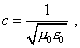(1)
 where c is the velocity of EM wave,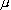0 is the magnetic constant and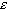0 is the dielectric constant. If these properties are attributed to the aether, irrespectively to its motion, the light velocity constancy will be true namely in the reference frame stationary as to the aether. As we showed in , when the reference frame moved relatively the aether, the wave propagation velocity varies; in this way the luminiferous aether was so unsuitable for Einstein. Thinking the aether present and having the wave pattern of EM excitation transmission (which needs not the aether's motion - it would be enough, it to be able to change locally its parameters), the 4 D metric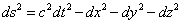(2)
 losses its validity. While Einstein had a greatest care to remain metric (2) true. Just the principle, "in SR, the effects of length and time reduction have a kinematic interpretation (not a dynamic - due to the interaction with ether)" [12, p. 331], due to which "there are lifted all questions, how to model physically such reduction" [ibidem], is the basis if geometrically abstract idyll that Einstein built. And not Abraham destroyed this idyll, he was right when pointed: when Einstein denied the light velocity constancy in the gravity field in passing to GR, he denied the relativity theory as the whole. And not Lenard destroyed who thought: "In physical consideration we can use two patterns which I call the first and second kind of patterns. Of the first-kind patterns says, for example, H. Weil, when he expresses all processes in equations. The second-kind patterns interpret the equations as the processes in space. I would prefer the second-kind patterns, while Einstein adheres to those of the first kind. In the patterns of second kind the ether is unavoidable. It always was one of the most important supplementary means to progress in the study of nature, and to abolish it would mean to abolish the second-kind patterns from the minds of all researchers of the nature" [13, p. 374]. The same, we cannot think, the cause that destroyed Einstein's idyll to be the following objection that the scales and clocks "are made, generally speaking, of many particles, which in a complicated way are affected by different power fields, so it is unclear, why just their behaviour has to be described by especially simple laws" [14, p. 124- 125]. The history of progress of Einstein's thought shows, it was Einstein himself who destroyed the idyll of SR, as he had to pass in GR development to the necessary physical associativeness of his abstract geometrical constructions. "As he progressed from the special theory of relativity to general theory and further towards the unified theory, Einstein's thought more and more clearly drew the relativity theory as the field theory. With it, there retained a requirement: the description of motion becomes meaningless, if it is not based on the image of some macroscopic body with a warranted metric - Euclidean either non- Euclidean, permanent either variable" [14, p. 167]. The further Einstein's thought penetrated to the area of field structures, the more, on one hand, Einstein denied his postulates of SR, and on the other hand he left that more or less sound basis which he built in frames of 4 D geometrisation of physical processes. The first sacrifice on this way was offered up when he denied the light velocity constancy in all inertial reference frames. He started so in his work of 1907 "On the relativity principle and its corollaries", in which he, in his typical manner to manipulate symbols, relates the time flowin a reference frame freely falling in the gravity field with the time flow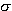in an inertial reference frame [15, p. 109]: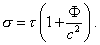Already in this formula we see a mistake that will stretch along all further derivation - Einstein used the symbol of light velocity, while in the next derivations he will come to its inconstancy. True, in this paper Einstein gives, as he says, an exact relationship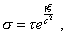but the symbol of light velocity remains in this formula. We have to add, the yielded relation of times is twice less than that necessary. So Einstein partially rewrites his derivation, the same unfoundedly doubling the numerator of the summand in brackets of the above formula. He shows the result in his paper "On the affection of gravity force on the light propagation" . In it, he considered the following model: "If the radiation emitted in an uniformly accelerated reference frame K' from S2 toward S1 had the frequency2 relatively the clock located in  S2 , then, having arrived to S1, it has the frequency not2 but larger frequency1 relatively the similar clock located there; this frequency in a first approximation will be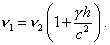(3)
 Indeed, if we introduce again a non-accelerated reference frame K0 , relatively which the reference frame K'  had a zero speed at the moment of light emission, S1 will have relatively K0 the speed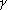(h/c) at the moment of light arriving to S1, where from, by force of Doppler principle, we immediately yield (3)" [16, p. 170]. In this derivation we can draw our attention to several points. Firstly, to study, how the clock rate will change, it is used an inertial frame K0 , in relation to which the clock rate in the uniformly accelerated frame K' is undetermined, and it is unknown at all, how can we determine it. Secondly, on one hand the light velocity variation is as if studied in an uniformly accelerated frame, but all computations are made in an inertial reference frame. So we see a clear duality. The term(h/c)  has been calculated for an inertial reference frame; to yield this term in this inertial reference frame, the light velocity has to be constant, lest to violate the laws of SR. While, as Einstein stated his problem, the light velocity varies just in a non-inertial reference frame. But in a non-inertial reference frame, the potential as such will be absent, since the system falls with an acceleration that is proportional to an attraction of material objects by a gravitating body. Hence, the formula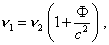(4)
 which Einstein yielded by way of substitution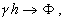(5)
 is true for the relation of inertial and non-inertial reference frames, if the non-inertial reference frame relates to the material bodies S1 and S2 that give to this frame an acceleration of free fall. While in the inertial reference frame, the light velocity cannot be considerably changed, in order to retain true the derivation of (4). Furthermore, accordingly to the statement of problem, the light source and receiver freely fall onto the gravitating centre which is located not between them. They move in one direct trajectory with some acceleration of free fall. But if the gravitating (point) centre is located between the source and receiver or somewhere aside, (4) will have basically other appearance. We have to understand (4) in this sense and in no other way. In this sense, Ives' rightness is fully corroborated on the operational system used by Einstein. "It correctly predicts the results of some selected operations, as factually, although it is not openly accepted, it was derived, proceeding from the ether acceptance and from the length and frequency reduction in the motion relatively the ether. Leaving without attention the question, which physical processes determine the applicability of this particular case, it gives no explanation to, what lays behind this specific choice of operations" [17, p. 156]. We can take whichever Einstein's derivation - and we will permanently encounter the only: artificial stipulations and trajectories, on whose basis Einstein makes his generalisations and extrapolates them onto absolutely other conditions and regularities.

Contents: / 1 / 2 / 3 / 4 / 5 / 6 / 7 / 8 / 9 / 10 / 11 / 12 / 13 / 14 / 15 / 16 / 17 /